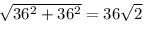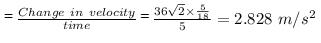Courses

# Test: Motion In Two Dimensions

## 5 Questions MCQ Test Physics Class 11 | Test: Motion In Two Dimensions

Description
This mock test of Test: Motion In Two Dimensions for Class 11 helps you for every Class 11 entrance exam. This contains 5 Multiple Choice Questions for Class 11 Test: Motion In Two Dimensions (mcq) to study with solutions a complete question bank. The solved questions answers in this Test: Motion In Two Dimensions quiz give you a good mix of easy questions and tough questions. Class 11 students definitely take this Test: Motion In Two Dimensions exercise for a better result in the exam. You can find other Test: Motion In Two Dimensions extra questions, long questions & short questions for Class 11 on EduRev as well by searching above.
QUESTION: 1

### A stone is thrown upwards with initial velocity of 20 m s-1, the height that stone will reach would be

Solution:

Initial velocity = u = 20 m/s
Final velocity = v = 0              [ at maximum height , v = 0 ]

Acceleration due to gravity(g)  in this case , is taken as negative.
This is because , when the direction of motion of object is opposite to "g" , then value of g is taken as -ve

hence ,

g = -9.8 m/s²

Let's use the formula :-

[h = height ]

v² -u² = 2gh

0² - 20² = 2*-9.8*h
-400 = -19.6h
h = -400/-19.6
= 20.408 m [ approximately ]

QUESTION: 2

### A particle has an initial velocity of 3i + 4j and an acceleration of 0.4i + 0.3j. Its speed after 10s is

Solution:

Use equation of kinematics: v = u + at

Initial Velocity, u = 3i + 4j

Acceleration, a = 0.4i + 0.3j

Time, t = 10s

v = (3i + 4j) + 10(0.4i + 0.3j)

v = (3i + 4j) + (4i + 3j)

v = 7i + 7j

|v| = √(72+72) = 7√2

QUESTION: 3

### A body sliding on a smooth inclined plane requires 4 seconds to reach the bottom starting from rest at the top. How much time does it take to cover one-fourth distance starting from rest at the top

Solution:

A body start from rest so, u=0

Let total distance covered = s

Let a body moves with accerlation = a

s=1/2 × a× t2

s=1/2× a × 42

s=8a

The one-fourth of total distance, s*  = 8a/4

=2a

s* = 1/2 × a × t2

2a / a=1/2 × t2

4 = t2

t=2

QUESTION: 4

A car travelling at 36km/h-1 due North turns West in 5 seconds and maintains the same speed. What is the acceleration of the car?

Solution:

Initial velocity of car = 36 km/hr due north
Final velocity of car = 36 km/hr due west

The magnitude of change in velocity(since velocity is a vector, so direction has to be taken into account)

AccelerationQUESTION: 5

Water drops fall at regular intervals from a tap which is 5m above the ground. The third drop is leaving the tap at the instant the first drop touches the ground. How far above the ground is the second drop at that instant

Solution:

Time taken by first drop to cover 5 cm, u = 0

⇒ h = 1/2 gt2

⇒ 5 = 1/2 x 10 x t2

⇒ t = 1 sec

Hence interval is 0.5 sec for each drop.

Now distance fallen by second drop in 0.5 sec

⇒ h1 = 1/2 gt2

= 1/2 x 10 x (0.5)2

= 5 x 0.25

= 1.25 m

Height above the ground ( of 2nd drop) = 5 - 1.25

= 3.75 m